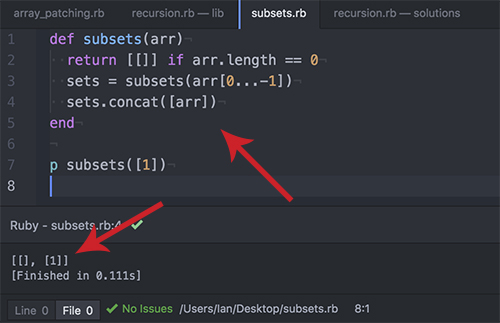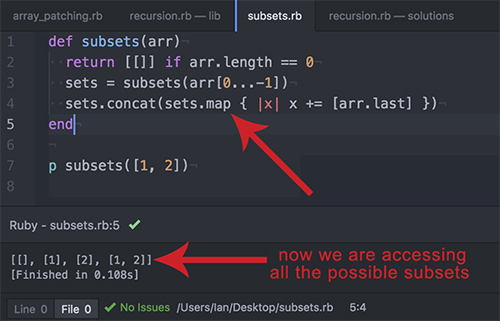# Learning Recursion in Ruby

“In order to understand recursion, you must first understand recursion.” – Source Unknown

## How it Clicked For Me

The key to understanding recursion is to get the base case.

To find the base case, define the easiest result.

Then think about the second easiest result.

As soon as you can get the first, second and third value, you have the solution for infinity.

It’s important to focus on the simplest case because when learning recursion, your mind can wander in the possibilities.

In the subsets example one can find a lot of strange patters when looking at all the subsets. Things like:

• Could we work on this based on array length?
• Is there a pattern in the order?
• Perhaps we could shuffle the arrays and ask if they are in the range?

These sorts of thoughts can go for infinity. I think this is what recursion is seen as such a challenging thing. There is a lot of room for thinkers to develop ideas that get more and more complex as the program gets longer.

In the end, recursion will win.

## Factorial Recursion

First off, here’s what we’re trying to find:
`4! == 4 * 3 * 2 * 1`
So we’re trying to write a ruby method that will do for our computer programs what the exclamation point (!) does for mathematicians.

Here’s how we write factorial in the Ruby programming language:
``` def factorial(n) return 1 if n == 1 n * factorial(n - 1) end ```

The factorial of 3 is 6. I’ve drawn it out in the hopes of explaining how the function works.
`factorial(3) == 3 * 2 * 1 == 6`## Talking Through Recursion

So in the above drawing you can see that we first call the function on 3. 3 isn’t equal to 1 so it goes to the next line of code and we multiply 3 by factorial (3 -1). So our next stack frame is created and recursively call the function.

Recursively call? What does that mean?

Recursively calling a function is calling the same function inside of it’s self.

We go another level deep seeking factorial(2). The first line of the function asks if 2 is equal to 1, and it’s not. Therefore, we move to the next line and multiply 2 times factorial (2 – 1). Factorial(2-1) is factorial(1).

Inside our third stack frame, we call factorial(1) but that only makes it to the first line of code because the function sees that 1 == 1. When 1 == 1 we return a 1 and the recursive loop stops.

When the loop stops, the return starts going back down the stack. The 1 we just returned goes up a level and multiplies by everything.

`1 * 2 * 3 == 6`

That’s how it works.

### What is Stack Overflow

In the above drawing, you can see that the function factorial is called three times when running this program. That means that it requires 3 stack levels.

This is how you can first understand the term stack overflow.

Ruby allows for more than 9,000 stack frames before it throws a stack overflow error.

Recursion gets more complex as the problems become more challenging. Here’s and example of a more complex example.

## Subset RecursionSo with this function we’re passing an array in and getting an array with every subset possible in that array.

The answer for subsets of an empty array would be an empty array.This is the easiest solution to the problem so we start by writing that.

`return [[]] if arr.length == 0`

Ok so the function gives the right answer if passed an empty array. The next step is to find out what happens if we pass an array with a single value.

`subsets() =(needs to be)= [[], ]`

So we essentially have to make an array with  and [].

No big deal right? We just add  and []… but first we need to get [].

`sets = subsets(arr[0...-1]) == []`

Great, now we just concat them together

`sets.concat([arr])`

So this gives us the right answer for the two most simple base cases.But our solution doesn’t give us all the base cases yet. It doesn’t work once we have more than one element in our array.So where is our solution failing right now? It’s failing because it isn’t counting the .

`subsets([1, 2]) == [[], , , [1, 2]]`

`subsets() == [[], ]`

`subsets() == [[]]`

We’re looking for is a function that add these subsets together. We know the tool needs to work in the recursive call or we will break the pattern. What is the problem right now?

The problem is that our function didn’t see  when calculating the sub arrays of [1, 2]. It failed to get the sub array possible in the second position. We know this problem will persist and get worse exponentially fast as we recurse through bigger data sets.

The way to make a note of all the possible sub arrays is to map out the function inside of the function.

`sets.concat(sets.map { |x| x += [arr.last] })`This is great! Now we can extend the tests to see if our recursive method works until infinity.`def subsets(arr)`

`return [[]] if arr.length == 0`

`sets = subsets(arr[0...-1])`

`sets.concat(sets.map { |x| x += [arr.last] })`

`end`

This function is incredibly hard to conceptualize when looking at a larger data set. But when you break it down to the core base cases, the reliable patterns emerge more explicitly. With more explicit data, solving the problem becomes something we can take one step at a time.

## Good Recursion Resources

Best video – Computerphile’s video

Visuals – Goolge Images

## 2 Replies to “Learning Recursion in Ruby”

1.Richard says:

Dont understand this line
“When the loop stops, the return starts going back down the stack. The 1 we just returned goes up a level and multiplies by everything.

1 * 2 * 3 == 6”

Why not just return ‘1’ when the loop stops? There is no instruction to do any multiplication once n reaches 1.

That’s how it works.

1.Ian Robinson says:

Right. Well put.

This site uses Akismet to reduce spam. Learn how your comment data is processed.